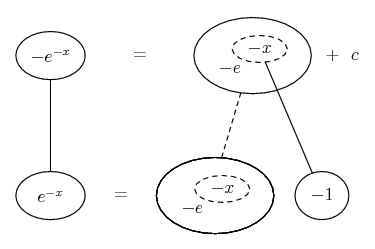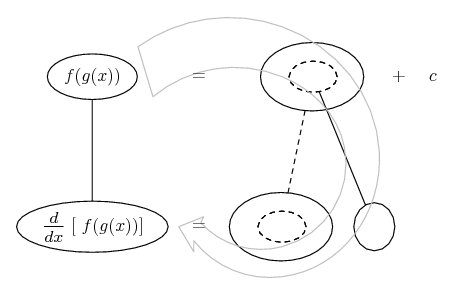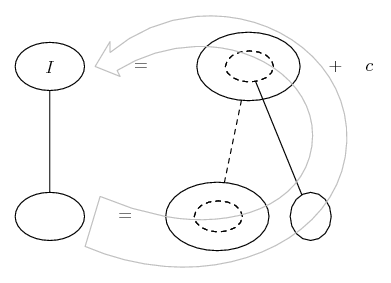# Thread: integration by substitution

1. ## integration by substitution

Question: evaluate the indefinite integral by using substitution
$\displaystyle \int \frac{e^x+1}{e^x}$
The answer key has $\displaystyle x-e^{-x}+C$

I tried the substitutions

1. $\displaystyle u=e^x\Rightarrow du=e^x dx$
2. $\displaystyle u=e^x+1\Rightarrow du=e^x dx$

1. doesn't really work so I think 2. is a better bet. I think I need to somehow get $\displaystyle du=\frac{dx}{e^x}$ but I don't know how.

2. $\displaystyle \displaystyle \frac{e^{x}+1}{e^x} = \frac{e^x}{e^x}+\frac{1}{e^x} = 1+\frac{1}{e^x} = 1+e^{-x}.$Originally Posted by Jskid1. doesn't really work so I think 2. is a better bet. I think I need to somehow get $\displaystyle du=\frac{dx}{e^x}$ but I don't know how.
Both subs should work. The first one gives:

$\displaystyle \displaystyle \frac{du}{dx} = e^x \Rightarrow dx = \frac{du}{e^x}$ so $\displaystyle \displaystyle \int\frac{e^x+1}{e^x}\;{dx} = \int\frac{e^x+1}{e^x\cdot e^x}}\;{du} = \int\frac{u+1}{u^2}\;{du} = ...$

3. Hello, Jskid!

$\displaystyle \text{Evaluate the inde{f}inite integral by using substitution:}$

. . $\displaystyle \displaystyle \int \frac{e^x+1}{e^x}\,dx$

Here's your second substitution . . .

Let $\displaystyle u \:=\:e^x+1 \quad\Rightarrow\quad e^x \:=\:u-1$
. . . . $\displaystyle x \:=\:\ln(u-1) \quad\Rightarrow\quad dx \:=\:\dfrac{du}{u-1}$

Substitute: .$\displaystyle \displaystyle \int\frac{u}{u-1}\cdot\frac{du}{u-1} \;=\;\int\frac{u\,du}{(u-1)^2}$

Partial fractions: .$\displaystyle \displaystyle \int\left(\frac{1}{u-1} + \frac{1}{(u-1)^2}\right)du \;=\;\int\frac{du}{u-1} + \int(u-1)^{-2}du$

Integrate: .$\displaystyle \ln|u-1| - (u-1)^{-1} + C$

Back-substitute: .$\displaystyle \ln|(e^x+1)-1| - \dfrac{1}{(e^x+1)-1} + C$

. . . . . . . . . . $\displaystyle \displaystyle =\;\ln|e^x| - \frac{1}{e^x} + C$

. . . . . . . . . . $\displaystyle =\;x - e^{-x} + C$

4.Originally Posted by TheCoffeeMachine$\displaystyle \displaystyle \frac{e^{x}+1}{e^x} = \frac{e^x}{e^x}+\frac{1}{e^x} = 1+\frac{1}{e^x} = 1+e^{-x}.$
I'm with you so far but I don't know how to integrate $\displaystyle e^{-x}$
let $\displaystyle u=e^{-x} \Rightarrow du=-e^{-x} dx$ what next?

@Soroban the textbook hasn't introducted partial fractions yet. Is there a way to solve this that doesn't require them?

5. Think about differentiation in reverse... You know the derivative of e^-x so work backwards

For reference $\displaystyle \displaystyle \int e^{ax} = \dfrac{1}{a}e^{ax}$

6.Originally Posted by JskidI'm with you so far but I don't know how to integrate $\displaystyle e^{-x}$
let $\displaystyle u=e^{-x} \Rightarrow du=-e^{-x} dx$ what next?
@Soroban the textbook hasn't introducted partial fractions yet. Is there a way to solve this that doesn't require them?
This problem has nothing to do with partial fractions.
Nothing more than basic, basic algebra tell us that $\displaystyle \dfrac{e^x+1}{e^x}=1+e^{-x}$.

Can you differentiate $\displaystyle -e^{-x}~?$

7.Originally Posted by e^(i*pi)For reference $\displaystyle \displaystyle \int e^{ax} = \dfrac{1}{a}e^{ax}$
This is not apperant to me. It looks simple but I don't actually know how it works, could someone elaborate?

8.Originally Posted by JskidThis is not apperant to me. It looks simple but I don't actually know how it works, could someone elaborate?
This a general result, commit it to memory, if you want a better understanding apply the chain rule to find the derivative of $\displaystyle \dfrac{1}{a}e^{ax}$

9. Use the substitution $\displaystyle ax = u \Rightarrow dx = \frac{du}{a}$.

10. Ok, I'll try to explain...Originally Posted by JskidI'm with you so far but I don't know how to integrate $\displaystyle e^{-x}$
let $\displaystyle u=e^{-x} \Rightarrow du=-e^{-x} dx$ what next?
Don't solve for $\displaystyle du$, solve for $\displaystyle dx$: we have $\displaystyle {dx} = -\frac{1}{e^{-x}}\;{du}.$

Your integral was $\displaystyle \int e^{-x} \;{dx}$, replacing the $\displaystyle dx$ with $\displaystyle -\frac{1}{e^{-x}}\;{du}$, we have:

$\displaystyle \int e^{-x} \;{dx} = \int e^{-x}\left(-\frac{1}{e^{-x}}\;{du}\right) = -\int\frac{e^{-x}}{e^{-x}}\;{du} = -\int~ 1~ \;{du} = -u+k$

But $\displaystyle u = e^{-x}$, so $\displaystyle \int e^{-x} \;{dx} = -e^{-x}+k.$

Do you understand now?

11. Also, just in case a picture helps...... where (as usual) ...represents the chain rule for differentiation - straight continuous lines differentiating down (integrating up) with respect to x, the straight dashed line similarly but with respect to the dashed balloon expression.

PS: I should have emphasised (and after Plato's comment below now can't resist)... read the diagram downwards to differentiate...and upwards to integrate..._________________________________________

Don't integrate - balloontegrate!

Balloon Calculus; standard integrals, derivatives and methods

Balloon Calculus Drawing with LaTeX and Asymptote!

12.Originally Posted by DefunktUse the substitution $\displaystyle ax = u \Rightarrow dx = \frac{du}{a}$.
I think that the method of u-substitution is most deleterious, non-productive idea that matheducators have foisted off on poor students honestly trying to learn to do anti-differentiation. It gives a false sense of understanding of the actual process. Now I do agree with the philosopher of mathematics Michael Resnik (I admit being a friend), he is known as a structurelist: mathematics is the science of pattern.

To find an anti-derivative, one needs to look at the pattern of derivatives.

13. So you would rather not teach substitution at all? [off topic, so I won't continue]

14. Thanks people I get it now.

#### Search Tags

integration, substitution